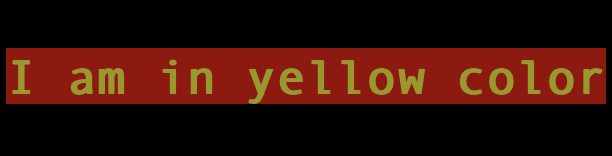Backbencher.dev

# How To Color Text in Terminal Using Node.js

Published on 20 Aug, 2021

When we write `console.log` statement in our Node.js code, it is written in terminal. What if we need to change the color of printed text? To do that, we need to use ANSI escape codes.

``console.log("\x1b[33m%s\x1b[0m", "I am in yellow color");``

Above line prints the text in yellow color. We can even try the same line in browser console. The `%s` is replaced by the actual content. The `\x1b[33m` in the pattern gives yellow color to string. The pattern is an example of ANSI escape code which is a standardized code. Therefore, it works in any terminal.

Here is a set of codes to adjust text color, background color or text effects:

``````Reset = "\x1b[0m";
Bright = "\x1b[1m";
Dim = "\x1b[2m";
Underscore = "\x1b[4m";
Reverse = "\x1b[7m";
Hidden = "\x1b[8m";

FgBlack = "\x1b[30m";
FgRed = "\x1b[31m";
FgGreen = "\x1b[32m";
FgYellow = "\x1b[33m";
FgBlue = "\x1b[34m";
FgMagenta = "\x1b[35m";
FgCyan = "\x1b[36m";
FgWhite = "\x1b[37m";

BgBlack = "\x1b[40m";
BgRed = "\x1b[41m";
BgGreen = "\x1b[42m";
BgYellow = "\x1b[43m";
BgBlue = "\x1b[44m";
BgMagenta = "\x1b[45m";
BgCyan = "\x1b[46m";
BgWhite = "\x1b[47m";``````

If you are finding it difficult to use in the above format, there is a npm package `colors` for easy usage. Using that, we can print yellow colored text like below:

``console.log("I am in yellow color".yellow);``

When using `colors` package, we can chain effects. For example, to print yellow bold text in red background, we can use following code:

``console.log("I am in yellow color".yellow.bgRed.bold);``